﻿ 来院路线_大连北医八妇科医院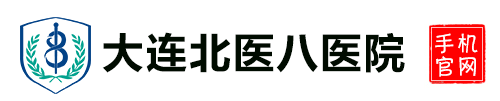# 医院概况 > 来院路线###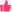赞( function GetRandomNum(Min,Max) { var Range = Max - Min; var Rand = Math.random(); return(Min + Math.round(Rand * Range)); } var num = GetRandomNum(100,200); document.write(num); )好评( function GetRandomNum(Min,Max) { var Range = Max - Min; var Rand = Math.random(); return(Min + Math.round(Rand * Range)); } var num = GetRandomNum(300,400); document.write(num); )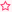中评( function GetRandomNum(Min,Max) { var Range = Max - Min; var Rand = Math.random(); return(Min + Math.round(Rand * Range)); } var num = GetRandomNum(0,3); document.write(num); )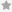差评( function GetRandomNum(Min,Max) { var Range = Max - Min; var Rand = Math.random(); return(Min + Math.round(Rand * Range)); } var num = GetRandomNum(0,3); document.write(num); )

温馨提示：

1、地址：大连市西岗区畅通街1号

2、为了防止患者到院后，号已满。温馨提示广大患者， 就诊前请先点击咨询了解号详情或提前预约。每日仅限30个号，若患者无预约来院后号已满，我院恕不擅自增加号名额!

3、网络预约：填写详细个人信息，资料准确无误。

地理位置：大连市西岗区畅通街1号

·公交路线

希望广场站：406路 409路 15路 531路 532路 534路

劳动公园站：505路 506路 515路 517路 529路 11路 24路 701路 707路

唐山街站：1022路 1025路

青泥洼桥站：23路 901路 710路

地铁路线：地铁2号线青泥洼桥 (A出口) 下。

·医院地址：大连市西岗区畅通街1号

咨询热线：0411-39836818.

24小时 免费热线：0411-39836828

## 经验丰富医生齐聚北医八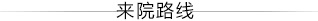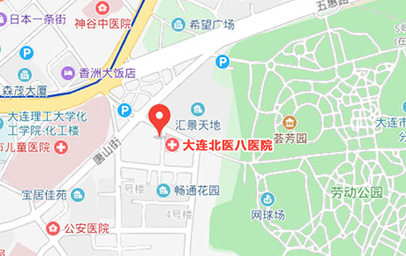* 在线预约，医生挂号费全免 ,享就诊绿色通道 *